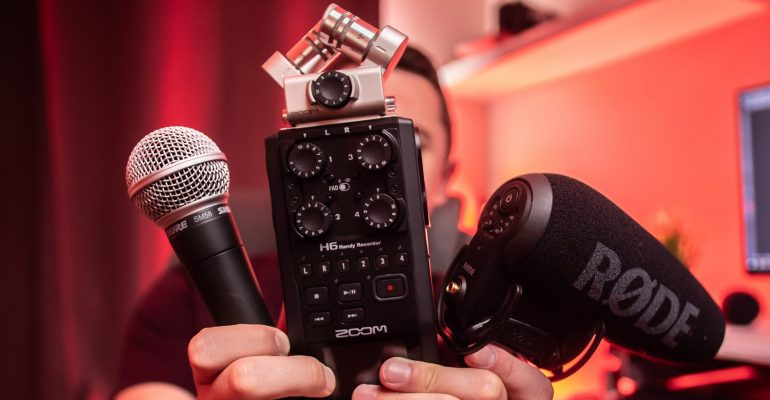Thanks to theidioms.com

## Open-Source Audio-based Data Science Projects (With Tutorials)# Open-Source Audio-based Data Science Projects (With Tutorials)The audio-based data science projects listed below are categorized in an experience-wise manner. All of these projects can be implemented using Python.

## Audio-based Data Science Projects

1. Speech Emotion Recognition – Tutorial

Our voices often reflect our emotions through tone and pitch. This project uses the same concept and attempts to recognize human emotion and affective states from his/her speech.

Summary of the steps involved in this project are:
2. Extract important features
3. Split the dataset into training and testing sets
4. Initialize MLPClassifier and train the model.

Full code for Speech Emotion Recognition in Python.

```import librosa
import soundfile
import os, glob, pickle
import numpy as np
from sklearn.model_selection import train_test_split
from sklearn.neural_network import MLPClassifier
from sklearn.metrics import accuracy_score

#Extract features (mfcc, chroma, mel) from a sound file
def extract_feature(file_name, mfcc, chroma, mel):
with soundfile.SoundFile(file_name) as sound_file:
sample_rate=sound_file.samplerate
if chroma:
stft=np.abs(librosa.stft(X))
result=np.array([])
if mfcc:
mfccs=np.mean(librosa.feature.mfcc(y=X, sr=sample_rate, n_mfcc=40).T, axis=0)
result=np.hstack((result, mfccs))
if chroma:
chroma=np.mean(librosa.feature.chroma_stft(S=stft, sr=sample_rate).T,axis=0)
result=np.hstack((result, chroma))
if mel:
mel=np.mean(librosa.feature.melspectrogram(X, sr=sample_rate).T,axis=0)
result=np.hstack((result, mel))
return result

#Emotions in the RAVDESS dataset
emotions={
'01':'neutral',
'02':'calm',
'03':'happy',
'05':'angry',
'06':'fearful',
'07':'disgust',
'08':'surprised'
}

#Emotions to observe
observed_emotions=['calm', 'happy', 'fearful', 'disgust']

#Load the data and extract features for each sound file
x,y=[],[]
for file in glob.glob("path_to_folder\\Actor_*\\*.wav"):
file_name=os.path.basename(file)
emotion=emotions[file_name.split("-")]
if emotion not in observed_emotions:
continue
feature=extract_feature(file, mfcc=True, chroma=True, mel=True)
x.append(feature)
y.append(emotion)
return train_test_split(np.array(x), y, test_size=test_size, random_state=9)

#Split the dataset

#Initialize the Multi Layer Perceptron Classifier
model=MLPClassifier(alpha=0.01, batch_size=256, epsilon=1e-08, hidden_layer_sizes=(300,), learning_rate='adaptive', max_iter=500)

#Train the model
model.fit(x_train,y_train)

#Predict for test score
y_pred=model.predict(x_test)

#Calculate the accuracy of our model
accuracy=accuracy_score(y_true=y_test, y_pred=y_pred)

#Print the accuracy
print("Accuracy: {:.2f}%".format(accuracy*100))```

2. Speech Recognizer – Video Tutorial, GitHub

Recognizing speech plays an important role in how we communicate with our devices. Technologies such as Google Assistant, Siri, Alexa heavily rely upon speech recognition. This project builds a simple speech recognizer to identify spoken digits. In this project, a neural network is built using the TFLearn high-level Tensorflow-based library which is then trained on a labeled dataset of spoken digits. Finally, it is tested on spoken digits. Watch the explanation by Siraj Rawal:

Full code for speech recognizer in python:

```from __future__ import division, print_function, absolute_import
import tflearn
import speech_data
import tensorflow as tf

learning_rate = 0.0001
training_iters = 300000  # steps
batch_size = 64

width = 20  # mfcc features
height = 80  # (max) length of utterance
classes = 10  # digits

batch = word_batch = speech_data.mfcc_batch_generator(batch_size)
X, Y = next(batch)
trainX, trainY = X, Y
testX, testY = X, Y #overfit for now

# Network building
net = tflearn.input_data([None, width, height])
net = tflearn.lstm(net, 128, dropout=0.8)
net = tflearn.fully_connected(net, classes, activation='softmax')
net = tflearn.regression(net, optimizer='adam', learning_rate=learning_rate, loss='categorical_crossentropy')
# Training

### add this "fix" for tensorflow version errors
col = tf.get_collection(tf.GraphKeys.TRAINABLE_VARIABLES)
for x in col:

model = tflearn.DNN(net, tensorboard_verbose=0)
while 1: #training_iters
model.fit(trainX, trainY, n_epoch=10, validation_set=(testX, testY), show_metric=True,
batch_size=batch_size)
_y=model.predict(X)
model.save("tflearn.lstm.model")
print (_y)
print (y)```

3. Music Instrument Classifier – Video Tutorial, GitHub

Classifying musical instruments might seem like a simple task for a human ear, but is far more complicated when it comes to computers. In this project, Seth Adams has built a musical instrument classifier is built which can identify 10 different musical instruments. It uses Convolutional Neural Networks and Recurrent Neural Networks for the classification purpose.

Watch the entire playlist.

4. Neural Network Voices – Video Explanation, GitHub

What if you could imitate a famous celebrity’s voice or sing like a famous singer? This project started with a goal to convert someone’s voice to the voice of a famous celebrity: Kate Winslet. Simply put, it’s voice style transfer. This project implements deep neural networks trained on more than 2 hours of audiobook sentences read by Kate Winslet. This project was made by Dabi Ahn and Kyubyong Park, and the following video by Siraj Rawal explains how the working of the project:

5. Music Unmixing – Written Tutorial, Colab Notebook

Music unmixing is the process of separating different instruments from a piece of music. It is one of the most interesting fields in the Audio Analysis. Music separation is a cornerstone problem for many applications in the entertainment industry. This tutorial was presented at EUSIPCO 2019 and it covers the topic from both, a theoretical perspective, as well as an interactive demonstration regarding how to implement the described ideas in practice.

## In Conclusion:

How many of the above projects have you tried? Do you have any recommendations for us to include in the above list? Let us know.

Also, if you are trying to start or advance your career in the field of Computer Vision, you might like this article on “Open-Source Computer Vision Projects (With Tutorials)“.

Are you interested in working with data? Get a free trial month of LinkedIn Learning and learn Data Science and Machine Learning courses using Python, R, and SQL from top instructors.

Enroll in any one of the courses from the following fields today on LinkedIn Learning and start your free month of learning:

Disclaimer: When you subscribe to LinkedIn Learning, we may earn a small commission as an affiliate. We love the platform so much so that we have been using it ourselves at The Click Reader.